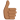# Complex numbers domain

• DottZakapa
In summary, the conversation discusses finding a domain with specific conditions for a complex number z=x+iy, where x and y are real numbers and |y-x|<=2 and |x|<=2. The participants discuss different approaches, such as using polar coordinates and graphing the region of interest, before realizing that the instructor had made a mistake in their calculation. Ultimately, the issue is resolved and the problem is solved using the original approach proposed by the OP.

#### DottZakapa

Homework Statement
i have to find this domain
Relevant Equations
complex numbers
i have to find such domain
z=x+iy , y,x∈ℝ , |y-x|<=2, |x|<=2

i'm confused with |y-x|<=2, how should i proceed ? with abs of x i am ok.

Have you tried to write ##x,y## is polar coordinates? Or start with reals: What does ##|x|<2## mean for real numbers?

##e^{-x}*e^{iy} = e^{-x+iy}## this?

DottZakapa said:
##e^{-x}*e^{iy} = e^{-x+iy}## this?
No. You have to resolve the norm, so you must represent the complex numbers ##x,y## somehow. Do you know how we can write them, in order to calculate ##|x|##?

i know that |z| = ##\sqrt {x^2+y^2}##
but i don't know how to handle this |y-x|

Last edited:
DottZakapa said:
i know that |z| = ##\sqrt {x^2+y^2}##
but i don't know how to handle this |y-x|
O.k. Polar coordinates would have been ##z=r\cdot e^{i\varphi}## and ##|z|=r##.
Anyway. In order to write ##|z| = \sqrt {x^2+y^2}## you had to write ##z=x+iy## first. This was a bad choice, as ##x,y## will be needed as complex numbers, so the letters are already occupied. You should have written ##z=a+ib## and ##|z|=\sqrt{a^2+b^2}.##

Now we have two complex variables ##x,y##. We first write them as ##x=a+ib## and ##y=c+id##. Can you calculate ##x-y## and ##|x-y|##? And you still have to answer post #2: Which domain is ##|a|<2## if ##a## is real?

so if x = a+ib and y= c+id then
y-x would be (c-a)+i(d-b) then the |y-x|= ##\sqrt {(c-a)^2+(d-b)^2}##

then
##\sqrt {(c-a)^2+(d-b)^2}<=2##=> ##(c-a)^2+(d-b)^2<=4##

DottZakapa said:
so if x = a+ib and y= c+id then
y-x would be (c-a)+i(d-b) then the |y-x|= ##\sqrt {(c-a)^2+(d-b)^2}##

then
##\sqrt {(c-a)^2+(d-b)^2}<=2##=> ##(c-a)^2+(d-b)^2<=4##
Yes, but you must be careful with squaring inequalities. You cannot go back easily. That's why I asked for the meaning of ##|a|<2##.

a is comprised between -2 and 2. isn't it?

Yes, and the same is true if ##Z=a+ib## is complex. However, the complex norm is defined to be positive, so here we get for ##z=a+ib## from ##|z|=|a+ib|=\sqrt{a^2+b^2}## that ##0\leq \sqrt{a^2+b^2}<2## must hold. Try to draw the points ##\{(a,b)\in \mathbb{R}\}^2\,|\,0\leq\sqrt{a^2+b^2}<2## in a coordinate system with axis ##a## and ##b##. And then figure out where ##z## has to be, if the coordinates were ##a## and ##ib##.

fresh_42 said:
This was a bad choice, as ##x,y## will be needed as complex numbers, so the letters are already occupied. You should have written ##z=a+ib## and ##|z|=\sqrt{a^2+b^2}.#

I disagree. $x \in \mathbb{R}$ and $y \in \mathbb{R}$ are defined by the OP's question as being the real and imaginary parts of $z \in \mathbb{C}$, and conditions are then placed upon $x$ and $y$ in order to restrict $z$ to a particular subset of $\mathbb{C}$. That is the natural way to interpret
DottZakapa said:
i have to find such domain
z=x+iy , y,x∈ℝ , |y-x|<=2, |x|<=2

So the OP is looking for $(x,y) \in \mathbb{R}^2$ such that $|y - x| \leq 2$ (and $|x| \leq 2$, but they already know how to deal with that).

So start with something easier. Take $C \in \mathbb{R}$ such that $|C| \leq 2$ and consider $y - x = C$. What does that look like?

•Delta2 and DottZakapa
pasmith said:
I disagree. $x \in \mathbb{R}$ and $y \in \mathbb{R}$ are defined by the OP's question as being the real and imaginary parts of $z \in \mathbb{C}$, and conditions are then placed upon $x$ and $y$ in order to restrict $z$ to a particular subset of $\mathbb{C}$. That is the natural way to interpret

So the OP is looking for $(x,y) \in \mathbb{R}^2$ such that $|y - x| \leq 2$ (and $|x| \leq 2$, but they already know how to deal with that).

So start with something easier. Take $C \in \mathbb{R}$ such that $|C| \leq 2$ and consider $y - x = C$. What does that look like?

i did try in such way, but the results of the instructor did not coincide. Going forward in the video, he made a mistake and corrected it. So problem sorted. Is like you say. thanks to anyone•WWGD
The region |y-x|<2 is clearly bounded by the lines y=x+2 and y=x-2, so sketch those. Superimpose the bounds for |x|<2.

•Delta2 and DottZakapa
thank you, as said above i have sorted the problem. the adopted procedure before posting the problem here was the same as @pasmith and you proposed. A dubdt raised for a discrepancy between my obtained result and the one of the instructor, which later on revealed to be a calculation mistake of the instructor.